# Math 8 - Systems of Linear Inequalities in Two Variables

21 Nov 2021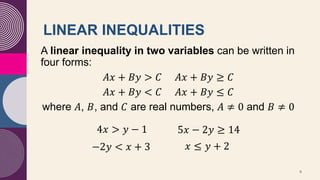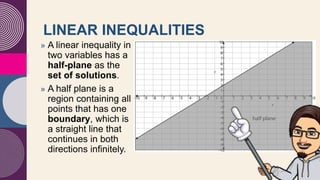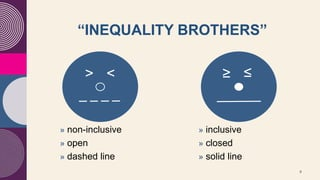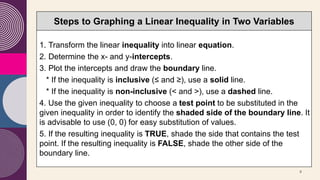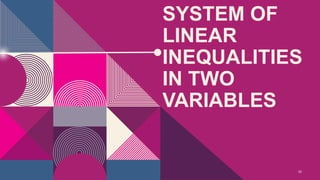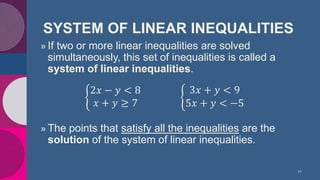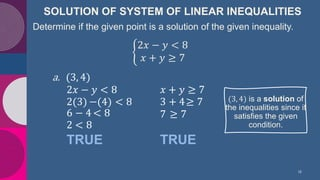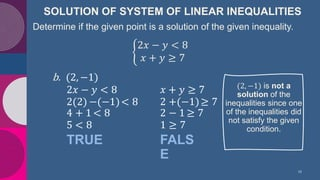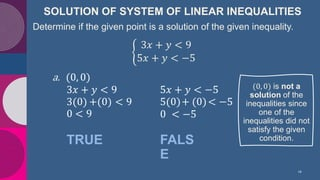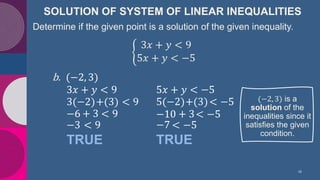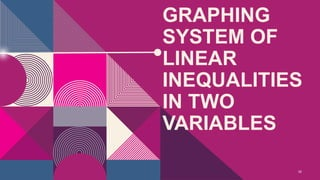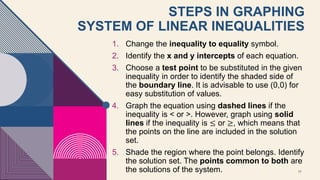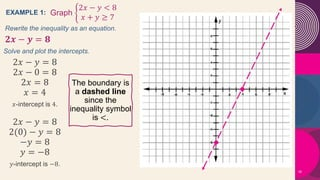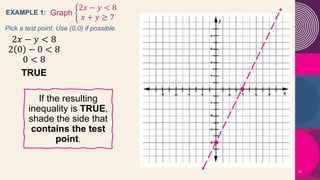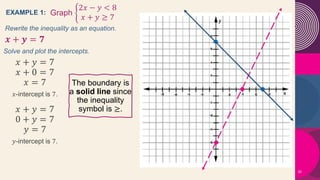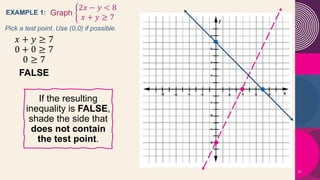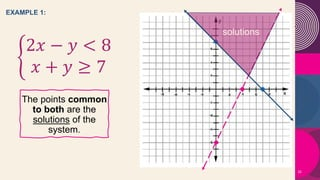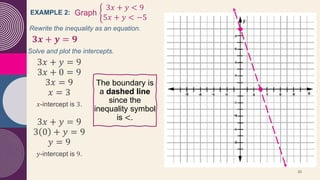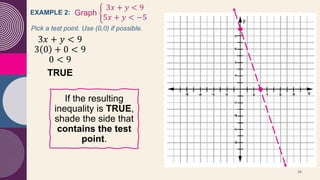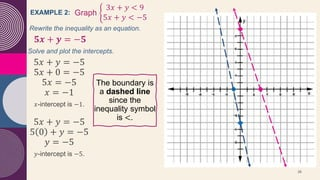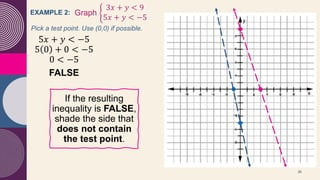1 sur 29

### Math 8 - Systems of Linear Inequalities in Two Variables

• 1. WELCOME to our Math Class LEARNING MATH IS fun-tastic MR. CARLO JUSTINO J. LUNA MALABANIAS INTEGRATED SCHOOL Angeles City
• 2. THE MATHEMATICIAN’S PRAYER Heavenly Father, thank You for all the blessings You gave unto us. Add joy to the world; Subtract evil from our lives, Multiply the good things for us; Divide the gifts and share them to others. Convert badness to goodness. Help us raise our needs to You. Extract the roots of immoralities and perform our different functions in life. Tell us all that life is as easy as math. Help us all to solve our problems. These we ask in Jesus’ name, the greatest mathematician who ever lived on earth, Amen! 2
• 3. MATHEMATICS 8 | Quarter 2 Week 1 SYSTEMS OF LINEAR INEQUALITIES in Two Variables MR. CARLO JUSTINO J. LUNA MALABANIAS INTEGRATED SCHOOL Angeles City
• 4. LEARNING COMPETENCIES M8AL-IIA-4 4 » Solve systems of linear inequalities in two variables » Graph systems of linear inequalities in two variables
• 6. LINEAR INEQUALITIES 6 A linear inequality in two variables can be written in four forms: 𝐴𝑥 + 𝐵𝑦 > 𝐶 𝐴𝑥 + 𝐵𝑦 ≥ 𝐶 𝐴𝑥 + 𝐵𝑦 < 𝐶 𝐴𝑥 + 𝐵𝑦 ≤ 𝐶 where 𝐴, 𝐵, and 𝐶 are real numbers, 𝐴 ≠ 0 and 𝐵 ≠ 0 4𝑥 > 𝑦 − 1 −2𝑦 < 𝑥 + 3 5𝑥 − 2𝑦 ≥ 14 𝑥 ≤ 𝑦 + 2
• 7. 7 » A linear inequality in two variables has a half-plane as the set of solutions. » A half plane is a region containing all points that has one boundary, which is a straight line that continues in both directions infinitely. LINEAR INEQUALITIES
• 8. 8 » non-inclusive » open » dashed line “INEQUALITY BROTHERS” > < ≥ ≤ » inclusive » closed » solid line
• 9. 9 Steps to Graphing a Linear Inequality in Two Variables 1. Transform the linear inequality into linear equation. 2. Determine the x- and y-intercepts. 3. Plot the intercepts and draw the boundary line. * If the inequality is inclusive (≤ and ≥), use a solid line. * If the inequality is non-inclusive (< and >), use a dashed line. 4. Use the given inequality to choose a test point to be substituted in the given inequality in order to identify the shaded side of the boundary line. It is advisable to use (0, 0) for easy substitution of values. 5. If the resulting inequality is TRUE, shade the side that contains the test point. If the resulting inequality is FALSE, shade the other side of the boundary line.
• 11. SYSTEM OF LINEAR INEQUALITIES 11 » If two or more linear inequalities are solved simultaneously, this set of inequalities is called a system of linear inequalities. » The points that satisfy all the inequalities are the solution of the system of linear inequalities. 2𝑥 − 𝑦 < 8 𝑥 + 𝑦 ≥ 7 3𝑥 + 𝑦 < 9 5𝑥 + 𝑦 < −5
• 12. SOLUTION OF SYSTEM OF LINEAR INEQUALITIES 12 Determine if the given point is a solution of the given inequality. 2𝑥 − 𝑦 < 8 𝑥 + 𝑦 ≥ 7 2𝑥 − 𝑦 < 8 a. (3, 4) 2(3) −(4) < 8 6 − 4 < 8 2 < 8 TRUE 𝑥 + 𝑦 ≥ 7 3 + 4≥ 7 7 ≥ 7 TRUE (3, 4) is a solution of the inequalities since it satisfies the given condition.
• 13. SOLUTION OF SYSTEM OF LINEAR INEQUALITIES 13 Determine if the given point is a solution of the given inequality. 2𝑥 − 𝑦 < 8 𝑥 + 𝑦 ≥ 7 2𝑥 − 𝑦 < 8 b. (2, −1) 2(2) −(−1)< 8 4 + 1 < 8 5 < 8 TRUE 𝑥 + 𝑦 ≥ 7 2 +(−1)≥ 7 2 − 1 ≥ 7 1 ≥ 7 FALS E (2, −1) is not a solution of the inequalities since one of the inequalities did not satisfy the given condition.
• 14. SOLUTION OF SYSTEM OF LINEAR INEQUALITIES 14 Determine if the given point is a solution of the given inequality. 3𝑥 + 𝑦 < 9 5𝑥 + 𝑦 < −5 3𝑥 + 𝑦 < 9 a. (0, 0) 3(0) +(0) < 9 0 < 9 TRUE 5𝑥 + 𝑦 < −5 5(0)+ (0)< −5 0 < −5 FALS E (0, 0) is not a solution of the inequalities since one of the inequalities did not satisfy the given condition.
• 15. SOLUTION OF SYSTEM OF LINEAR INEQUALITIES 15 Determine if the given point is a solution of the given inequality. 3𝑥 + 𝑦 < 9 5𝑥 + 𝑦 < −5 3𝑥 + 𝑦 < 9 b. (−2, 3) 3(−2)+(3) < 9 −6 < 9 TRUE 5𝑥 + 𝑦 < −5 5(−2)+(3)< −5 −10 < −5 TRUE + 3 −3 < 9 + 3 < −5 −7 (−2, 3) is a solution of the inequalities since it satisfies the given condition.
• 17. 17 STEPS IN GRAPHING SYSTEM OF LINEAR INEQUALITIES 1. Change the inequality to equality symbol. 2. Identify the x and y intercepts of each equation. 3. Choose a test point to be substituted in the given inequality in order to identify the shaded side of the boundary line. It is advisable to use (0,0) for easy substitution of values. 4. Graph the equation using dashed lines if the inequality is < or >. However, graph using solid lines if the inequality is ≤ or ≥, which means that the points on the line are included in the solution set. 5. Shade the region where the point belongs. Identify the solution set. The points common to both are the solutions of the system.
• 18. EXAMPLE 1: 18 Graph 2𝑥 − 𝑦 < 8 𝑥 + 𝑦 ≥ 7 𝟐𝒙 − 𝒚 = 𝟖 Rewrite the inequality as an equation. 2𝑥 − 𝑦 = 8 2𝑥 − 0 = 8 2𝑥 = 8 𝑥 = 4 𝑥-intercept is 4. Solve and plot the intercepts. 2𝑥 − 𝑦 = 8 2(0) − 𝑦 = 8 −𝑦 = 8 𝑦 = −8 𝑦-intercept is −8. The boundary is a dashed line since the inequality symbol is <.
• 19. EXAMPLE 1: 19 Graph 2𝑥 − 𝑦 < 8 𝑥 + 𝑦 ≥ 7 2𝑥 − 𝑦 < 8 2 0 − 0 < 8 0 < 8 TRUE Pick a test point. Use (0,0) if possible. If the resulting inequality is TRUE, shade the side that contains the test point.
• 20. EXAMPLE 1: 20 Graph 2𝑥 − 𝑦 < 8 𝑥 + 𝑦 ≥ 7 𝒙 + 𝒚 = 𝟕 Rewrite the inequality as an equation. 𝑥 + 𝑦 = 7 𝑥 + 0 = 7 𝑥 = 7 𝑥-intercept is 7. Solve and plot the intercepts. 𝑥 + 𝑦 = 7 0 + 𝑦 = 7 𝑦 = 7 𝑦-intercept is 7. The boundary is a solid line since the inequality symbol is ≥.
• 21. EXAMPLE 1: 21 Graph 2𝑥 − 𝑦 < 8 𝑥 + 𝑦 ≥ 7 𝑥 + 𝑦 ≥ 7 0 + 0 ≥ 7 0 ≥ 7 FALSE Pick a test point. Use (0,0) if possible. If the resulting inequality is FALSE, shade the side that does not contain the test point.
• 22. EXAMPLE 1: 22 2𝑥 − 𝑦 < 8 𝑥 + 𝑦 ≥ 7 The points common to both are the solutions of the system. solutions
• 23. 23 EXAMPLE 2: Graph 3𝑥 + 𝑦 < 9 5𝑥 + 𝑦 < −5 𝟑𝒙 + 𝒚 = 𝟗 Rewrite the inequality as an equation. 3𝑥 + 𝑦 = 9 3𝑥 + 0 = 9 3𝑥 = 9 𝑥 = 3 𝑥-intercept is 3. Solve and plot the intercepts. 3𝑥 + 𝑦 = 9 3 0 + 𝑦 = 9 𝑦 = 9 𝑦-intercept is 9. The boundary is a dashed line since the inequality symbol is <.
• 24. 24 EXAMPLE 2: Graph 3𝑥 + 𝑦 < 9 5𝑥 + 𝑦 < −5 3𝑥 + 𝑦 < 9 3 0 + 0 < 9 0 < 9 TRUE Pick a test point. Use (0,0) if possible. If the resulting inequality is TRUE, shade the side that contains the test point.
• 25. 25 EXAMPLE 2: Graph 3𝑥 + 𝑦 < 9 5𝑥 + 𝑦 < −5 𝟓𝒙 + 𝒚 = −𝟓 Rewrite the inequality as an equation. 5𝑥 + 𝑦 = −5 5𝑥 + 0 = −5 5𝑥 = −5 𝑥 = −1 𝑥-intercept is −1. Solve and plot the intercepts. 5𝑥 + 𝑦 = −5 5 0 + 𝑦 = −5 𝑦 = −5 𝑦-intercept is −5. The boundary is a dashed line since the inequality symbol is <.
• 26. 26 EXAMPLE 2: Graph 3𝑥 + 𝑦 < 9 5𝑥 + 𝑦 < −5 5𝑥 + 𝑦 < −5 5 0 + 0 < −5 0 < −5 FALSE Pick a test point. Use (0,0) if possible. If the resulting inequality is FALSE, shade the side that does not contain the test point.
• 27. 27 EXAMPLE 2: 3𝑥 + 𝑦 < 9 5𝑥 + 𝑦 < −5 The points common to both are the solutions of the system. solutions
• 28. 28 ASYNCHRONOUS / SELF- LEARNING ACTIVITIES Answer the following: Quarter 2 Week 2 • What’s In • What’s More (items 1 & 3 only) Google Forms link • https://forms.gle/PoMK1dQUjyRoKT5Q7
• 29. 29 MATHEMATICS 8 Quarter 2 Week 2 THANK You MR. CARLO JUSTINO J. LUNA MALABANIAS INTEGRATED SCHOOL Angeles City